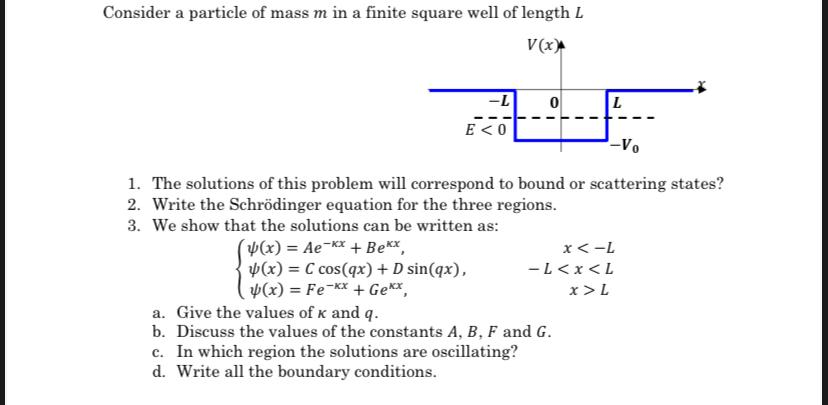# (Solved): Consider a particle of mass $$m$$ in a finite square well of length $$L$$ 1. The solutions of ...Consider a particle of mass $$m$$ in a finite square well of length $$L$$ 1. The solutions of this problem will correspond to bound or scattering states? 2. Write the Schrödinger equation for the three regions. 3. We show that the solutions can be written as: $\left\{\begin{array}{lr} \psi(x)=A e^{-\kappa x}+B e^{\kappa x}, & x<-L \\ \psi(x)=C \cos (q x)+D \sin (q x), & -LL \end{array}\right.$ a. Give the values of $$\kappa$$ and $$q$$. b. Discuss the values of the constants $$A, B, F$$ and $$G$$. c. In which region the solutions are oscillating? d. Write all the boundary conditions.

We have an Answer from Expert